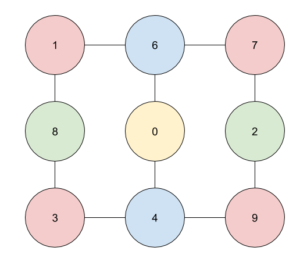One of the many lovely things about Big MathsJam is that I’ve found My People - I’ve made several very dear friends there, introduced others to the circle, and get to stay in touch with other maths fans through the year. It’s golden.

Adam Atkinson is one of those dear friends, and one of the ways he stays in touch is to send me puzzles. This one, a question Alex Golec has used to interview people at Google, made me struggle for a bit, then grin in smug triumph - which is a very MathsJam reaction.

### The problem

If you can’t be bothered clicking the link, the problem is to determine how many different phone numbers you can dial if you can only make $n$ knight’s moves on a standard keypad. Nothing artificial about that, no sir.

Golec’s post goes into some detail about how to solve it with code, which might be fine for Google, but it’s not the MathsJam way ((It’s totally OK to solve things with code at MathsJam. It’s just harder to be smug about.))

I did it a different way. Below the line be spoilers.

My first move was to draw a graph showing how the different numbers connect:Number 5, it turns out, doesn’t allow a move anywhere else, so it’s not on the graph.

The nine digits can be grouped into four classes according to the kinds of neighbours they have: Corner digits (1, 3, 7 and 9), Triples (4 and 6), Middles (2 and 8) and Zero (well, 0).

I’m going to set up four functions here: $C(n)$, $T(n)$, $M(n)$ and $Z(n)$, each being how many numbers you can dial starting from a digit in each of the four classes.

If you start at a Corner, you can immediately move to a Triple or to a Middle, which means $C(n) = T(n-1) + M(n-1)$.

If you start at a Triple, you can move to either of two Corners, or to Zero: $T(n) = 2C(n-1) + Z(n-1)$.

Starting at a Middle, your next move is to either of two Corners: $M(n) = 2C(n-1)$.

And starting at Zero, your next move is to either Triple: $Z(n) = 2T(n-1)$.

We also have, by definition, $C(0) = T(0) = M(0) = Z(0) = 1$.

### I spy a recurrence relation!

We have four linked recurrence relations - and what with this being Google and all, that looks like a linear algebra problem. We can set it up as a matrix:

$\left( \begin{array}{c} C(n) \\ T(n) \\ M(n) \\ Z(n) \end{array} \right) = \left( \begin{array}{cccc} 0 & 1 & 1 & 0 \\ 2 & 0 & 0 & 1 \\ 2 & 0 & 0 & 0 \\ 0 & 2 & 0 & 0 \end{array} \right) \left( \begin{array}{c} C(n-1) \\ T(n-1) \\ M(n-1) \\ Z(n-1) \end{array} \right)$

If I say $\bb{X}(n) = \left( \begin{array}{c} C(n) \\ T(n) \\ M(n) \\ Z(n) \end{array} \right)$ and $\bb{M} = \left( \begin{array}{cccc} 0 & 1 & 1 & 0 \\ 2 & 0 & 0 & 1 \\ 2 & 0 & 0 & 0 \\ 0 & 2 & 0 & 0 \end{array} \right)$, that can be written as:

$\bb{X}(n) = \bb{M} \cdot \bb{X}(n-1)$, with $\bb{X}(0) = \left( \begin{array}{c} 1 \\ 1 \\1 \\1 \end{array} \right)$.

But that means $\bb{X}(n) = \bb{M}^n \left( \begin{array}{c} 1 \\ 1 \\ 1 \\ 1 \end{array} \right)$, which can be computed quickly for any value of $n$.